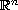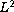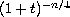Electron. J. Diff. Eqns., Vol. 2005(2005), No. 125, pp. 1-13.

### Decay of solutions to equations modelling incompressible bipolar non-newtonian fluids Bo-Qing Dong

Abstract:
This article concerns systems of equations that model incompressible bipolar non-Newtonian fluid motion in the whole space. Using the improved Fourier splitting method, we prove that a weak solution decays in thenorm at the same rate asas the timeapproaches infinity. Also we obtain optimalerror-estimates for Newtonian and Non-Newtonian flows.

Submitted March 28, 2005. Published November 7, 2005.
Math Subject Classifications: 35B40, 35Q35, 76A05.
Key Words: Decay; bipolar non-Newtonian fluids; Fourier splitting method.

Show me the PDF file (259K), TEX file, and other files for this article.Bo-Qing Dong School of Mathematical Sciences Nankai University Tianjin 300071, China email: bqdong@mail.nankai.edu.cn